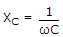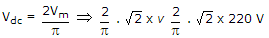# Electronics and Communication Engineering - Analog Electronics

11.

Which component is allowed to pass through it by a choke filter?

 A. Dc B. Ac C. Both dc and ac D. Only high frequency ac

Explanation:

XL is zero for dc.

12.

In an amplifier the stray capacitances assume impedance at low frequencies

 A. True B. False

Explanation:

Stray capacitances assume importance at high frequencies because.

13.

In CE amplifier the base current is very high.

 A. True B. False

Explanation:

Base current is small.

14.

In the graphical analysis of CE amplifier circuit, the upper end of load line is called

 A. saturation point B. cut off point C. Q point D. none of the above

Explanation:

Current is maximum at saturation point.

15.

A full wave rectifier supplies a load of 1 kΩ. The a.c. Voltage applied to the diodes is 220 - 0 - 220 Volts rms. If diode resistance is neglected, then Average d.c. Voltage

 A. 220 V B. 200 V C. 198 V D. 0 volts.